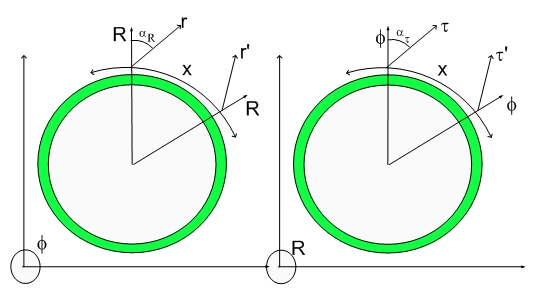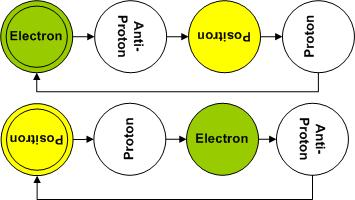# Spontaneous Breakdown of Symmetry...Spontaneous Breakdown of Symmetry and The Great Achievement of Achieving True Supersymmety

Needless to say, this theory is aiming at being a Great Achievement of Some Sort Someday...:)

My prior blog was about the how Gallileo Gallilei Equivalence Principle - equating Inertial Mass to Gravitational Mass - threw off scientists into believing that there was an Spontaneous Breakdown of Symmetry between the Four Fundamental Fources of Physics..:)

Here, I should repeat the Fantastic Four: Gravitation, Electromagnetism, Strong and Weak Forces.

It should be clear to anyone who reads this blog that the Strong Force was replaced by simple local Fabric of Space deformational energy (Force). Weak force, which is responsible for the beta decay, was eliminated when I changed the paradigm for Nuclear Chemistry to Nonlinear Hadronics (similar to Nonlinear Optics).

If you are familiar with the symmetries associated with these forces, the Strong Force requires an infinite force at infinite distance while the others require Infinite Forces at Zero Distances. This means that the supersymmetric force would require infinite force on both extremes...:)

By eliminating the Strong and Weak forces by substituting them by infinitely simpler paradigms, I eliminated this symmetry requirement. The Strong Force is a direct byproduct of the Standard Model. The introduction of spurious Quarks which couldn't ever be seen resulted in the introduction of spurious forces that act in a crazy way... They GLUED things together to explain a null result or failed experiment.

I am sure someone is going to ask me how could the results of the Standard Model be so precise and yet be wrong...:) Well, well... the first question to ask is if the Standard Model has parameters..>:) Given enough degrees of freedom one can solve any problem to any precision.

My theory has no degrees of freedom. In lieu of a degree of freedom, it has the fundamental dilator paradigm, which places the 4D Masses of the Proton and Electron equal to the 3D Mass of an Hydrogen Atom...:) or half of the 4DMass of an Hydrogen Atom...

One might say that this is a parameter. I beg to differ. It is a paradigm..:) one that goes hand in hand with the Lightspeed Expanding Hyperspherical Universe (another paradigm which explains 3D Universe expansion without Dark Energy, Dark Matter etc), Hubble constant etc.
Together with these two paradigm, I placed the Quantum Lagrangian Principle stating that a fundamental dilator would only dilate in phase with surrounding dilaton fields, that is, a dilator wouldn't try to expand space in a point when everyone else is trying to compress that region of space...:) Another simple paradigm that can be shown in a simple experiment similar to the one where sand patterns are created in a vibrating drum...:)

The remaining asymmetry is due to the different masses of Protons and Electrons...:) If they had exactly the same mass, one could formulate an electromagnetic theory based upon positive and negative masses - in analogy to Gravitation (formulated in terms of neutral masss).

The simple solution to the problem was to rewrite Gauss and Newton's Laws in a 4D Spatial Manifold where a shockwave Universe traverses a Cartesian 4D Manifold at the speed of light...:)

The reformulated Gauss and Newton's Laws are similar to the 3D ones. The only difference are in the constants... and in the fact that in 4D your are twice as Fat!!!!!!

According to the Fundamental Dilator Paradigm, Gravitational 4D Masses of Electrons and Protons are proportional to the coherently aggregated 4D Displacement volumes associated with the Fundamental Dilator cycle. This is because the transitions occur in phase with the generated waves. This is because the source of the dilaton field travels at the same speed as the dilaton field itself...:)

Remember, the fundamental dilator is a lightspeed traversing shape-shifting displacement volume (metric modulation) that changes the distances between any two points diametrically positioned around it. That volume displacement can be an small dilation (electron), a big dilation (antiproton), a small compression (positron) or a big compression (proton).Since all these phases occur as the dilator travels radially at the speed of light on the center of a horospherical reference frame, all the waves generated by each phase add in phase, thus it is the same effect as if one had an electron-positron dilator and a proton-antiproton dilator in their appropriate phases.

For this reason, I say that the 4DMass of an electron is the same as the 4D mass of a proton. I equate this to the atomic mass of a hydrogen atom. This means that an hydrogen atom will have the 4DMass of two Hydrogen Atoms..>:) or weight twice as much in 4D as they weight in 3D...:)

Notice that each phase of the fundamental dilator coherence is not a particle. Each one of the four fundamental particles (proton, antiproton, electron and positron) are represented by the same ever-repeating coherence, differing only by which phase is in phase with the shockwave 3D Universe.

This means that all equations of electromagnetism can be derived (and were derived in my papers) from an equation identical to Newton's Gravitation Law. The only difference is that one uses the mass of one hydrogen atom when calculating the number of dilators (electron or protons) per 4D Kilogram...:)

Without going into too many details here, it is late...:), it should be clear how the concept of the Fundamental Dilator eliminates the last asymmetry in the Fantastic Two..:) the last two standing forces..:)

The concept of Inertia, which puzzled mankind forever was explained in simple terms as the "area" of deformation in a Stress/Strain paradigm. This simple linear equation replaces Newton's Second Law (F=m.a) with a tremendous advantage. It doesn't introduce the concepts of Mass and Force..:) It keeps it all geometrical...:) as they say on the streets..:) Keep it Geometrical..:)

It is disappointing to many, me included, that inertia didn't keep the nonlocal nature proposed by Mach. It would be nice if we all could be part of the whole in a cryptic manner like the one expressed in - the part is equal to the whole..:) etc..

Sometimes it is nice that our philosophical mind is let down...:) One doesn't really need to have the part equal to the whole - the duality supplanted by a trinity..etc... or four huge turtles holding the Universe in place....or the Celestial Spheres being replaced by Celestial Hyperspheres...:)

Just take what life gives you and make the most of it...:)

This theory illuminates the path to an Infinite and Clean Source of Energy, The Promise of Space Travel .... it takes away some crazy ideas like wormhole travels or going into a Black Holes or whatever you might had concocted to look really smart in your latest paper...:)

Take Off Your Kazoos and Let Cellebrate The Arrival of Our 12,000 reader.

Thanks for taking the time and spreading the word about this work. It has been done with the belief that Ideas Are Important ...:)

They should always face scrutiny...:) I've just scrutinized Gallileo, Newton and Everyone who wasted time talking about wormhole travel..>:) Just make enough progress to go around the Solar System first...:)

By the way, scrutiny is always welcome...:) Leave your comments or email me directly ... The email adress is in my profile.

Cheers,

MP
Currently unrated

### New Comment

required

required (not published)

optional

required